Anúncio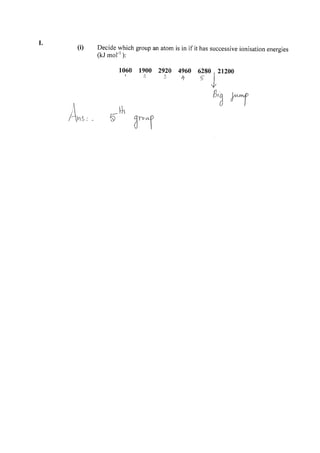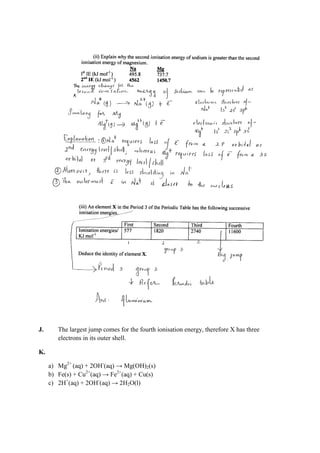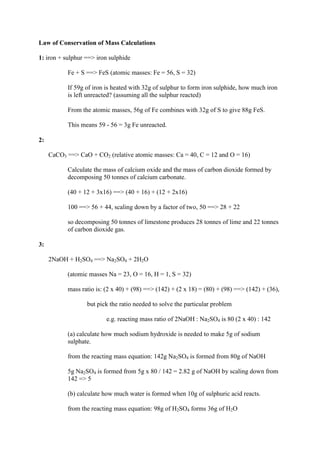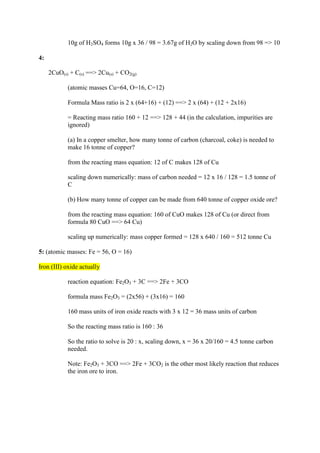AnúncioPróximos SlideShares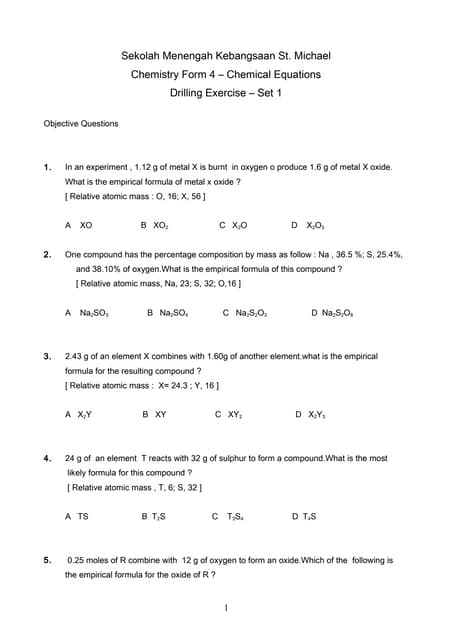Bengkel 1 chemical equation
Carregando em ... 3
1 de 5
Anúncio

### Tutorial 2 part 2 answer key

1. I.
2. J. The largest jump comes for the fourth ionisation energy, therefore X has three electrons in its outer shell. K. a) Mg2+ (aq) + 2OH- (aq) → Mg(OH)2(s) b) Fe(s) + Cu2+ (aq) → Fe2+ (aq) + Cu(s) c) 2H+ (aq) + 2OH- (aq) → 2H2O(l)
3. Law of Conservation of Mass Calculations 1: iron + sulphur ==> iron sulphide Fe + S ==> FeS (atomic masses: Fe = 56, S = 32) If 59g of iron is heated with 32g of sulphur to form iron sulphide, how much iron is left unreacted? (assuming all the sulphur reacted) From the atomic masses, 56g of Fe combines with 32g of S to give 88g FeS. This means 59 - 56 = 3g Fe unreacted. 2: CaCO3 ==> CaO + CO2 (relative atomic masses: Ca = 40, C = 12 and O = 16) Calculate the mass of calcium oxide and the mass of carbon dioxide formed by decomposing 50 tonnes of calcium carbonate. (40 + 12 + 3x16) ==> (40 + 16) + (12 + 2x16) 100 ==> 56 + 44, scaling down by a factor of two, 50 ==> 28 + 22 so decomposing 50 tonnes of limestone produces 28 tonnes of lime and 22 tonnes of carbon dioxide gas. 3: 2NaOH + H2SO4 ==> Na2SO4 + 2H2O (atomic masses Na = 23, O = 16, H = 1, S = 32) mass ratio is: (2 x 40) + (98) ==> (142) + (2 x 18) = (80) + (98) ==> (142) + (36), but pick the ratio needed to solve the particular problem e.g. reacting mass ratio of 2NaOH : Na2SO4 is 80 (2 x 40) : 142 (a) calculate how much sodium hydroxide is needed to make 5g of sodium sulphate. from the reacting mass equation: 142g Na2SO4 is formed from 80g of NaOH 5g Na2SO4 is formed from 5g x 80 / 142 = 2.82 g of NaOH by scaling down from 142 => 5 (b) calculate how much water is formed when 10g of sulphuric acid reacts. from the reacting mass equation: 98g of H2SO4 forms 36g of H2O
4. 10g of H2SO4 forms 10g x 36 / 98 = 3.67g of H2O by scaling down from 98 => 10 4: 2CuO(s) + C(s) ==> 2Cu(s) + CO2(g) (atomic masses Cu=64, O=16, C=12) Formula Mass ratio is 2 x (64+16) + (12) ==> 2 x (64) + (12 + 2x16) = Reacting mass ratio 160 + 12 ==> 128 + 44 (in the calculation, impurities are ignored) (a) In a copper smelter, how many tonne of carbon (charcoal, coke) is needed to make 16 tonne of copper? from the reacting mass equation: 12 of C makes 128 of Cu scaling down numerically: mass of carbon needed = 12 x 16 / 128 = 1.5 tonne of C (b) How many tonne of copper can be made from 640 tonne of copper oxide ore? from the reacting mass equation: 160 of CuO makes 128 of Cu (or direct from formula 80 CuO ==> 64 Cu) scaling up numerically: mass copper formed = 128 x 640 / 160 = 512 tonne Cu 5: (atomic masses: Fe = 56, O = 16) Iron (III) oxide actually reaction equation: Fe2O3 + 3C ==> 2Fe + 3CO formula mass Fe2O3 = (2x56) + (3x16) = 160 160 mass units of iron oxide reacts with 3 x 12 = 36 mass units of carbon So the reacting mass ratio is 160 : 36 So the ratio to solve is 20 : x, scaling down, x = 36 x 20/160 = 4.5 tonne carbon needed. Note: Fe2O3 + 3CO ==> 2Fe + 3CO2 is the other most likely reaction that reduces the iron ore to iron.
Anúncio